Chapter 2 Biofilm Growth and Development
Section 2 Biofilm Growth and Development
Page 2 Microbial Growth

## Microbial Growth

As discussed in the blue/green levels of this chapter microbial cells use nutrients for growth, energy production and product formation as indicated in the following expression;

Nutrients + microbial cells > cell growth + energy + reaction products

Consider the operation of the "Batch" system shown in  Figure 1. This container initially contains a known growth substrate concentration S. The container is well mixed and therefore the dissolved oxygen concentration O2 does not become a limiting factor for microbial growth. Initially a known concentration X of viable microbial cells (i.e. inoculum) is added to the container and, with time, growth substrate S is utilized for cell growth. We therefore over time will observe a decrease in S (negative dS/dt) and a corresponding  increase in X (positive dX/dt).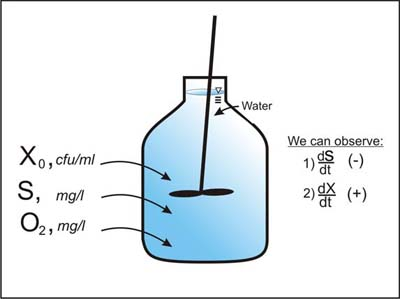Permissions

A. Cunningham, Center for Biofilm Engineering, Montana State Univeristy, Bozeman, MT

Figure 1. Microbial growth and substrate utilization in a well mixed batch container.

A conceptual plot of microbial cell concentration vs time for the batch system is called a growth curve, as shown in Figure 2.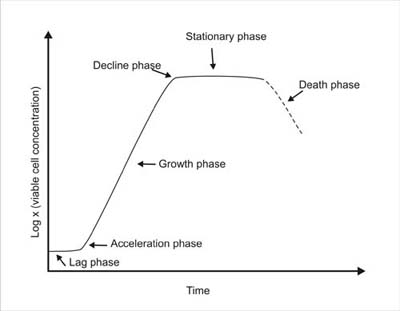Permissions

A. Cunningham, Center for Biofilm Engineering, Montana State Univeristy, Bozeman, MT

Figure 2. Typical growth curve for a batch system.
By plotting the log of viable cell concentration, X, with time, five distinct phases of the growth curve can be identified; 1) the lag phase which occurs immediately after inoculation and persists until the cells have acclimated to their new environment, 2) exponential growth phase, during which time cell growth proceeds at an exponential rate (indicated by a straight line on the semi-log plot), 3) a deceleration phase, when essential nutrients are depleted or toxic products begin to accumulate, 4) a stationary phase during which time the net cell growth is approximately zero, and 5) death phase where some cells loose viability or are destroyed by lysis.

### Microbial Growth Kinetics

During the lag phase dX/dt and dS/dt are essentially zero. However as exponential growth phase begins it is possible to measure dX/dt and dS/dt values which are very useful for defining important microbial kinetic parameters. Using corresponding observations of dS/dt and dX/dt obtained just after the onset of exponential growth phase in Figure 2 we can compute the yield coefficient YXS and the specific growth rate µ as:

#### Yield coefficient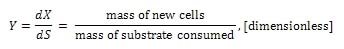(1)

#### Specific growth rate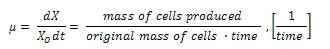(2)

The yield coefficient, commonly referred to as the substrate-to-biomass yield, is used to convert between cell growth rate dX/dt and substrate utilization rate dS/dt. The yield coefficient and the specific growth rate used to develop three types of microbial growth kinetic relationships; Monod , first order ,and zero order kinetics.

### Monod Kinetics

The batch experiment shown in Figure 1 can be repeated by varying initial substrate concentration S over a wide range of values—resulting in observation of individual µ values which correspond to each substrate concentration. An arithmetic plot of µ vs S will exhibit the general behavior shown in Figure 3.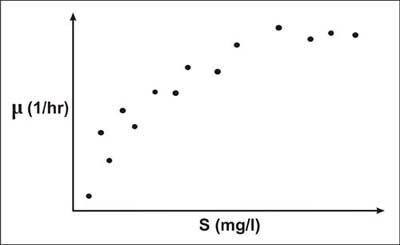Permissions

A. Cunningham, Center for Biofilm Engineering, Montana State Univeristy, Bozeman, MT

Figure 3. Specific growth rate plotted respect to initial substrate concentration in a batch system.

The most widely used expression for describing specific growth rate as a function of substrate concentration is attributed to Monod (1942, 1949). This expression is: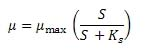(3)

Figure 4.  Shows conceptually how the Monod equation is fit to the observed substrate and  specific growth rate data in Figure 3. In Figure 4 it is seen that µmax is the maximum specific growth rate observed and KS  is the substrate concentration corresponding to 1/2 µmax.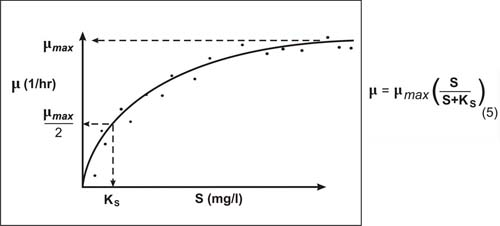Permissions

A. Cunningham, Center for Biofilm Engineering, Montana State Univeristy, Bozeman, MT

Figure 4. Monod Equation fit to observed data.

### Monod Kinetics

By combining equations 2 and 3 we can write the following expression for time-rate-of-change of biomass: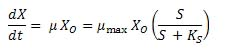(4)

Similarly, by combining equations 1 and 3 we can write an expression for substrate utilization rate.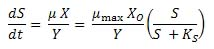(5)

### First Order Kinetics

Equation 5 describes the Monod kinetic relationship for substrate utilization. From Figure 4 it can be seen if  S <<  KS , Equation 5 can be approximated  as: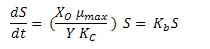(6)

Equation 6 describes the condition where substrate utilization is proportional to substrate concentration (i.e. first order with respect to S).

### Zero Order Kinetics

Likewise if  S >> KS Equation 5 can be approximated as: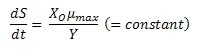(7)

Equation 7 describes the condition where substrate utilization rate is a constant (i.e.  zero order with respect to S).